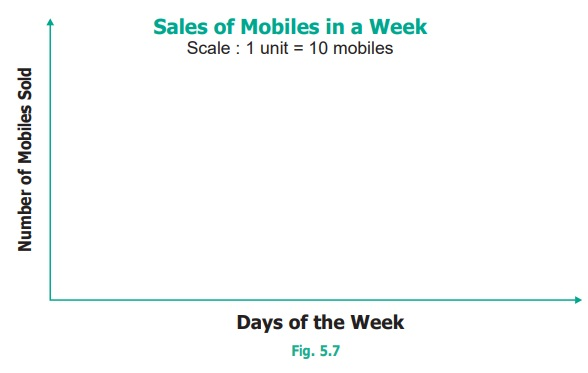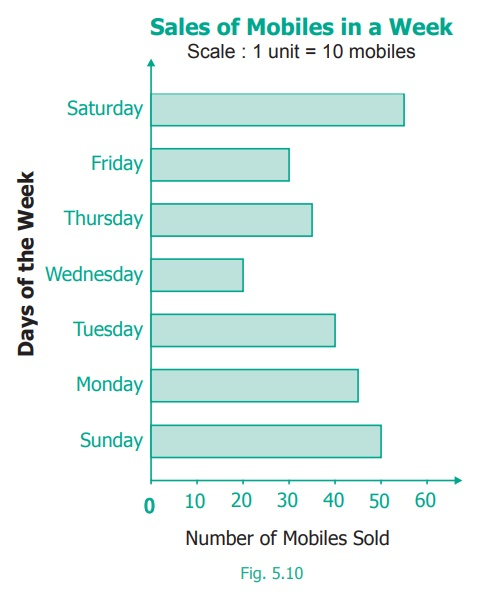Home | | Maths 6th Std | Representation of data using Bar Graph

# Representation of data using Bar Graph

A Bar graph consists of equally − spaced parallel bars (Horizontal or Vertical) whose lengths / heights are proportional to the number of items given.

Representation of data using Bar Graph

Ragavi’s father is a mobile shop owner. She finds the following data of sale of mobiles in a week.Note

A Bar graph consists of equally − spaced parallel bars (Horizontal or Vertical) whose lengths / heights are proportional to the number of items given.

1. Drawing Bar-Graph

Step1. Draw two lines which are perpendicular to each other. One of them is horizontal and the other one is vertical.Step2. A suitable title (Sale of mobiles in a week) is given. The lines are labelled (Horizontal line → Days of the week; vertical line → Number of mobiles sold).Step3. A suitable scale is chosen. The scale used is stated on the graph.Step4. Let the vertical line start from 0 and the values of information are marked at equal distances in same increments.Step5.For each information vertical bars are drawn on the horizontal line. They are labelled by respective information (as Monday, Tuesday… Sunday).This graph is called as Vertical Bar Graph.

The corresponding horizontal Bar Graph will look like this:

Sales of Mobiles in a Week

Scale: 1 unit = 10 mobilesThink

Why are these important?

● The width of each bar is same.

● The spaces between any two bars are also the same.

2. Interpreting the Bar Graph

The data from the above Bar Graph (Fig. 5.10) can be easily interpreted and analyzed as follows.

The maximum number of mobiles were sold on Sunday (55).

The minimum number of mobiles were sold on Thursday (20).

The total number of mobiles sold in the week (50+45+40+20+35+30+55 = 275).

The number of mobiles sold on a particular day (for example: on Friday is 35, etc.,).

Activity

Collect different data from Newspapers, Magazines, etc. and interpret them using Bar graphs.

Example 5.4Study the above Bar graph and answer the following questions.

(i) Which activity is followed by maximum number of students?

(ii) How many students in all, spend their time on solving puzzles?

(iii) The total number of students who follow either reading stories or reading their subjects is __________.

(iv) The activity followed by minimum number of students is __________.

(v) The number of students who took part in reading comics is __________.

Solution

(i) ‘Reading stories’ is followed by maximum number of students.

(ii) 7 students spend their time to work out solving puzzles.

(iii) 8 + 4 = 12 students spend their time on reading.

(iv) ‘Studying subject’ is followed by minimum number of students.

(v) 5 students spend their time on reading comics.

Think

Can you use